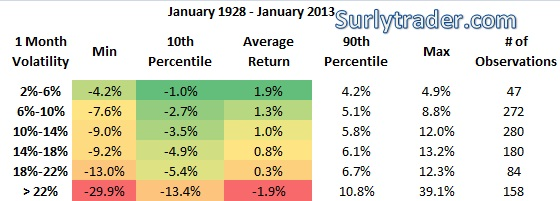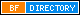Categories:

# Market Volatility and Returns

Do returns dictate volatility or does volatility dictate returns?  It might seem like a silly question, but one that is worth spending a bit of time on.  If market returns actually followed normal distributions, then we might expect that there are as many up days as down days and upside deviation equals downside deviation.  Unfortunately, the distribution of returns is rather non-normal with a larger number of negative returns and a lot of small positive returns.  You can read about option pricing and equity return distributions at a popular old post, “Do Black Swans Negate Option Premiums“.

The question for today is whether volatility necessarily means that returns are negative.  For this little bit of analysis, we will look at the S&P 500 daily returns going back to 1928.  The data is calculated into calendar month statistic sets and then the sets are grouped by realized volatility buckets:Monthly returns for low volatility months are higher than high volatility months

The data is pretty consistent across all metrics.  Low realized volatility generally corresponds to higher average returns whereas high realized volatility generally corresponds to lower average returns.  We can also see that (as expected and almost by definition) the dispersion between the highest and lowest returns increases as the volatility increases.

The question that arises is whether this has practical implications.  It has almost become an industry accepted fact that no one can predict the equity market.  Has it also become a fact that no one can predict future market volatility?  A quick google search would show you that there is quite a lot of literature that suggests volatility is much easier to predict.